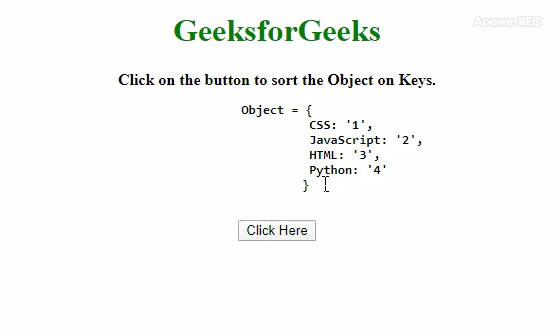# How to Sort/Order keys in JavaScript objects ?

Given an object and the task is to sort the JavaScript Object on the basis of keys. Here are a few of the most used techniques discussed with the help of JavaScript.

Approach 1: By using .sort() method to sort the keys according to the conditions specified in the function and get the sorted keys in the array. To copy the whole object to that temporary variable in the order of keys in a key array(in sorted fashion) and delete the original object will make a temporary variable. After that, we just need to copy the temporary object to the original object and return it.

• Example 1: This example implements the above approach.

 ` ` `<``html``> ` ` `  `<``head``> ` `    ``<``title``> ` `        ``How to Sort/Order keys ` `        ``in JavaScript objects ? ` `    `` ` `     `  `    ``<``style``> ` `        ``body { ` `            ``text-align: center; ` `        ``} ` `        ``h1 { ` `            ``color: green; ` `        ``} ` `        ``#geeks { ` `            ``font-size: 20px;  ` `            ``font-weight: bold; ` `        ``} ` `    `` ` ` ` ` `  `<``body``> ` `    ``<``h1``>GeeksforGeeks ` `     `  `    ``<``b``> ` `        ``Click on the button to sort  ` `        ``the Object on Keys. ` `        ``<``br``> ` `        ``<``pre``>Object = { ` `                    ``CSS: '1', ` `                    ``JavaScript: '2', ` `                    ``HTML: '3', ` `                    ``Python: '4' ` `                    ``} ` `        `` ` `    `` ` `     `  `    ``<``button` `onclick``=``"gfg_Run()"``> ` `        ``Click Here ` `    `` ` `     `  `    ``<``p` `id``=``"geeks"``> ` `     `  `    ``<``script``> ` `        ``var el_down = document.getElementById("geeks"); ` `         `  `        ``var GFG_Object = { ` `            ``CSS: '1', ` `            ``JavaScript: '2', ` `            ``HTML: '3', ` `            ``Python: '4' ` `        ``}; ` ` `  `        ``// Sorted keys are obtained in 'key' array ` `        ``function sortKeys(obj_1) { ` `            ``var key = Object.keys(obj_1) ` `            ``.sort(function order(key1, key2) { ` `                ``if (key1 < ``key2``) return -1; ` `                ``else if (key1 > key2) return +1; ` `                ``else return 0; ` `            ``});  ` `             `  `            ``// Taking the object in 'temp' object ` `            ``// and deleting the original object. ` `            ``var temp = {}; ` `             `  `            ``for (var i = 0; i < ``key.length``; i++) { ` `                ``temp[key[i]] = obj_1[key[i]]; ` `                ``delete obj_1[key[i]]; ` `            ``}  ` ` `  `            ``// Copying the object from 'temp' to  ` `            ``// 'original object'. ` `            ``for (var ``i` `= ``0``; i < key.length; i++) { ` `                ``obj_1[key[i]] = temp[key[i]]; ` `            ``}  ` `            ``return obj_1; ` `        ``} ` ` `  `        ``function gfg_Run() { ` `            ``el_down.innerHTML` `= ``JSON` `            ``.stringify(sortKeys(GFG_Object)); ` `        ``} ` `    `` ` ` ` ` `  ` `

• Output:• Approach 2: In this approach .sort() method sort the keys alphabetically then we have to use .reduce() method on these sorted keys. Then call an anonymous function inside reduce() method with 2 variables(emptyObject and key). Now one by one put the key and value pair in the emptyObject and return it.

• Example 2: This example implements the above approach.

 ` ` `<``html``> ` ` `  `<``head``> ` `    ``<``title``> ` `        ``How to Sort/Order keys ` `        ``in JavaScript objects ? ` `    `` ` `     `  `    ``<``style``> ` `        ``body { ` `            ``text-align: center; ` `        ``} ` `        ``h1 { ` `            ``color: green; ` `        ``} ` `        ``#geeks { ` `            ``font-size: 20px;  ` `            ``font-weight: bold; ` `        ``} ` `    `` ` ` ` ` `  `<``body``> ` `    ``<``h1``>GeeksforGeeks ` `     `  `    ``<``b``> ` `        ``Click on the button to sort  ` `        ``the Object on Keys.<``br``> ` `        ``<``pre``>Object = { ` `                ``CSS: '1', ` `                ``JavaScript: '2', ` `                ``HTML: '3', ` `                ``Python: '4' ` `            ``} ` `        `` ` `    `` ` `     `  `    ``<``button` `onclick``=``"gfg_Run()"``> ` `        ``Click Here ` `    `` ` `     `  `    ``<``p` `id``=``"geeks"``> ` `     `  `    ``<``script``> ` `        ``var el_down = document.getElementById("geeks"); ` `     `  `        ``var GFG_Object = { ` `            ``CSS: '1', ` `            ``JavaScript: '2', ` `            ``HTML: '3', ` `            ``Python: '4' ` `        ``}; ` ` `  `        ``function gfg_Run() { ` `             `  `            ``// Getting the keys of JavaScript Object. ` `            ``Modified_Object = Object.keys(GFG_Object)  ` `             `  `            ``// Sort and calling a method on ` `            ``// keys on sorted fashion. ` `            ``.sort().reduce(function(Obj, key) {  ` `                     `  `                ``// Adding the key-value pair to the ` `                ``// new object in sorted keys manner ` `                ``Obj[key] = GFG_Object[key];  ` `                ``return Obj;  ` `            ``}, {}); ` `            ``el_down.innerHTML =  ` `                    ``JSON.stringify(Modified_Object); ` `        ``} ` `    `` ` ` ` ` `  ` `

• Output:My Personal Notes arrow_drop_upCheck out this Author's contributed articles.

If you like GeeksforGeeks and would like to contribute, you can also write an article using contribute.geeksforgeeks.org or mail your article to contribute@geeksforgeeks.org. See your article appearing on the GeeksforGeeks main page and help other Geeks.

Please Improve this article if you find anything incorrect by clicking on the "Improve Article" button below.